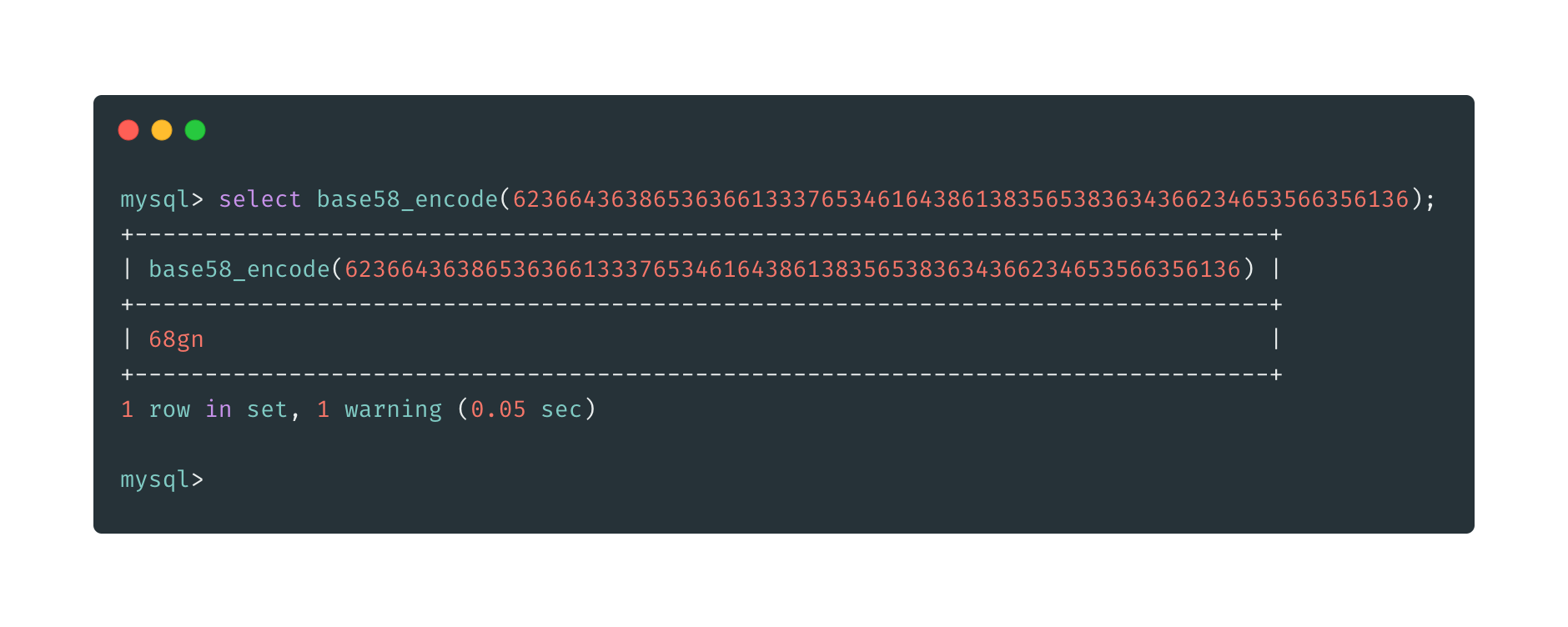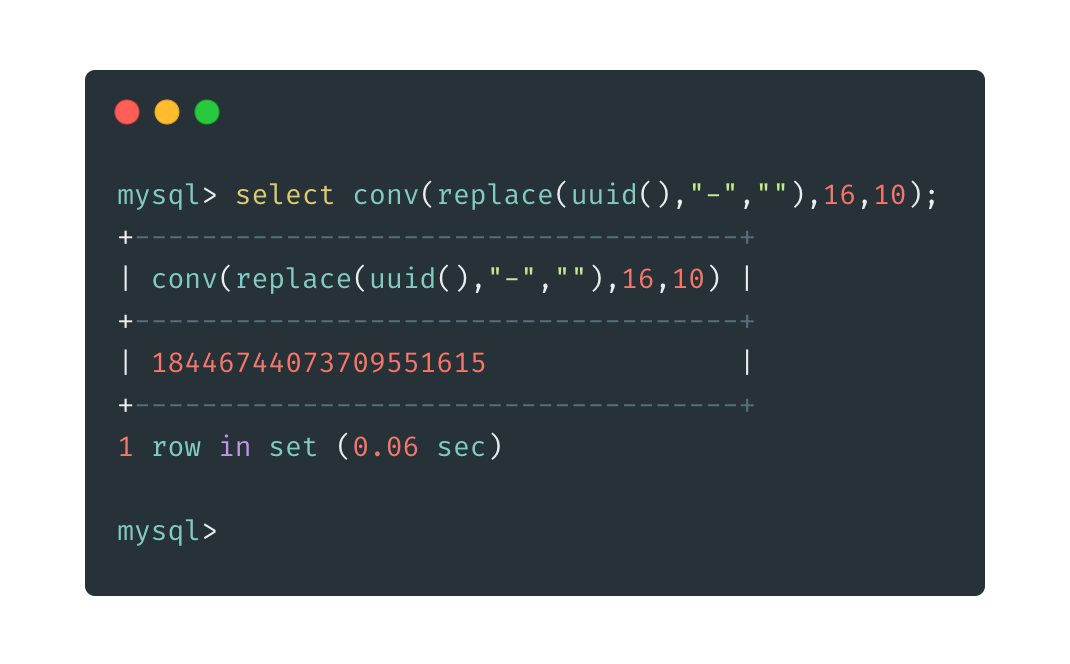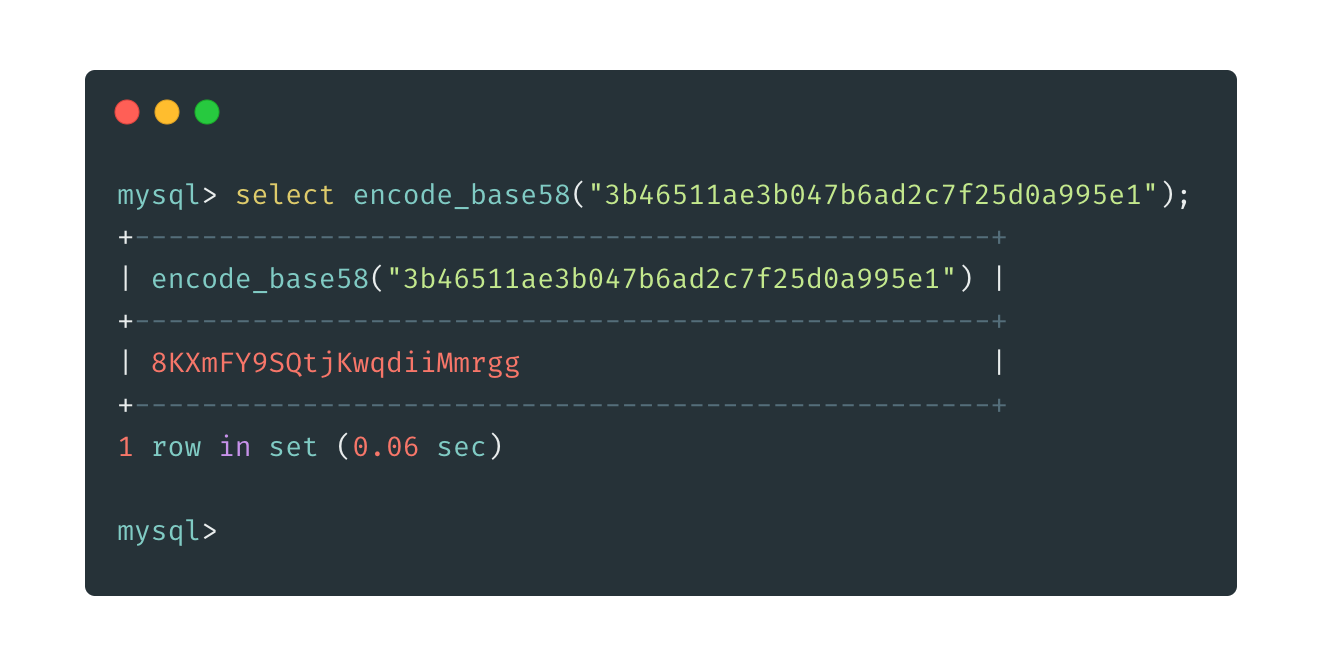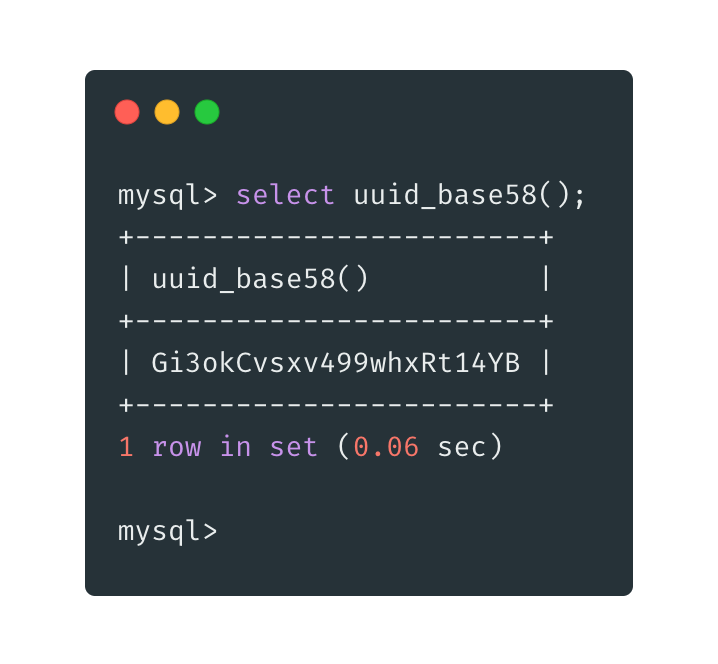# Base-58 Encoding really big numbers in MySQL (5.6>)(128 bit)

Encoding numbers in base-58 via MySQL is fairly straightforward until you start overflowing integer types. Basically as soon as you reach a 65bit number. If you search for base-58 encoding in MySQL there is not a lot of info overall. Micah Walter has a clean function called base58_encode that works well until you toss in anything larger than a BIGINT. It overflows:If you are using base-58 encoding to create unique identifiers you run into problems sending in really large hash values. A UUID is a perfect example at 128bits of data to cover the entire UUID. A UUID’s 128bits overflows the BIGINT quickly and you end up with the infamous -1 max value: 18446744073709551615.In MySQL you can use DECIMAL types to work around this and can create an encoder that will encode really large numbers. Decimal in MySQL is not stored with an FPU but is stored as characters. However, can you can still do `MOD()` and mathematics on it which is all we need for encoding. The challenge becomes dealing with the least significant 64-bits and then the most significant 64-bits of the incoming value.

Here is how I encode really big numbers using DECIMAL instead of BIGINT to provide a wider result. This solutions uses a MySQL function and is using the Bitcoin alphabet:

``````-- Change delimiter so that the function body doesn't end the function declaration
DELIMITER //
CREATE FUNCTION base58_encode(hexInput VARCHAR(32))
RETURNS TEXT
BEGIN
DECLARE alphabet CHAR(58);
DECLARE base10 DECIMAL(65);
DECLARE base58 TEXT;
DECLARE inputLength INT;
DECLARE remainder INT;

SET inputLength = LENGTH(hexInput);

IF (inputLength > 32) THEN
SIGNAL SQLSTATE '40004' SET MESSAGE_TEXT = "Input has a 32 character maximum";
END IF;

-- Convert the lower 64bits from base-16 to base-10
SET base10 = CAST(
CONV(SUBSTRING(hexInput, -LEAST(16, inputLength)), 16, 10)
AS DECIMAL(65)
);
-- Convert the most significant 64bits from base-16 to base-10
IF inputLength > 16 THEN
SET base10 = base10 + (
18446744073709551616 * CAST(
CONV(SUBSTRING(hexInput, 1, inputLength - 16), 16, 10)
AS DECIMAL(65)
)
);
END IF;

-- Encode in Base58
SET alphabet = "123456789ABCDEFGHJKLMNPQRSTUVWXYZabcdefghijkmnopqrstuvwxyz";
SET base58 = "";
WHILE base10 > 0 DO
SET remainder = base10 % 58;
SET base58 = CONCAT(
SUBSTRING(alphabet, remainder + 1, 1),
base58
);
SET base10 = FLOOR(base10 / 58);
END WHILE;

return base58;
END;
//

-- Switch back the delimiter
DELIMITER ;
``````

## Using it to created unique IDs

I typically build solutions with public facing identifiers that are built from UUIDs. For this I use ssvac’s solution for v4 UUIDs and I combine it into a single function called uuid_base58 - this way I can create IDs automatically at the dB level.

Here I create a few functions:

• `uuid_v4_no_dashes()` which is Ssvac’s solution with out the dashes
• `base58_encode(VARCHAR)` which is the encoder from above
• `uuid_base58()` which simplifies both calls
``````-- Change delimiter so that the function body doesn't end the function declaration
DELIMITER //

CREATE FUNCTION uuid_v4_no_dashes()
RETURNS CHAR(36)
BEGIN
-- Generate 8 2-byte strings that we will combine into a UUIDv4
SET @h1 = LPAD(HEX(FLOOR(RAND() * 0xffff)), 4, '0');
SET @h2 = LPAD(HEX(FLOOR(RAND() * 0xffff)), 4, '0');
SET @h3 = LPAD(HEX(FLOOR(RAND() * 0xffff)), 4, '0');
SET @h6 = LPAD(HEX(FLOOR(RAND() * 0xffff)), 4, '0');
SET @h7 = LPAD(HEX(FLOOR(RAND() * 0xffff)), 4, '0');
SET @h8 = LPAD(HEX(FLOOR(RAND() * 0xffff)), 4, '0');

SET @h4 = CONCAT('4', LPAD(HEX(FLOOR(RAND() * 0x0fff)), 3, '0'));

-- 5th section first half-byte can only be 8, 9 A or B
SET @h5 = CONCAT(HEX(FLOOR(RAND() * 4 + 8)),

-- Build the complete UUID
RETURN LOWER(CONCAT(
@h1, @h2, @h3, @h4, @h5, @h6, @h7, @h8
));
END
//
-- Switch back the delimiter
DELIMITER ;

-- Change delimiter so that the function body doesn't end the function declaration
DELIMITER //
CREATE FUNCTION uuid_base58()
RETURNS CHAR(22) DETERMINISTIC
BEGIN
return base58_encode(uuid_v4_no_dashes());
END
//

-- Switch back the delimiter
DELIMITER ;

-- Change delimiter so that the function body doesn't end the function declaration
DELIMITER //
CREATE FUNCTION base58_encode(hexInput VARCHAR(32))
RETURNS TEXT
BEGIN
DECLARE alphabet CHAR(58);
DECLARE base10 DECIMAL(65);
DECLARE base58 TEXT;
DECLARE inputLength INT;
DECLARE remainder INT;

SET inputLength = LENGTH(hexInput);

IF (inputLength > 32) THEN
SIGNAL SQLSTATE '40004' SET MESSAGE_TEXT = "Input has a 32 character maximum";
END IF;

-- Convert the lower 64bits from base-16 to base-10
SET base10 = CAST(
CONV(SUBSTRING(hexInput, -LEAST(16, inputLength)), 16, 10)
AS DECIMAL(65)
);
-- Convert the most significant 64bits from base-16 to base-10
IF inputLength > 16 THEN
SET base10 = base10 + (
18446744073709551616 * CAST(
CONV(SUBSTRING(hexInput, 1, inputLength - 16), 16, 10)
AS DECIMAL(65)
)
);
END IF;

-- Encode in Base58
SET alphabet = "123456789ABCDEFGHJKLMNPQRSTUVWXYZabcdefghijkmnopqrstuvwxyz";
SET base58 = "";
WHILE base10 > 0 DO
SET remainder = base10 % 58;
SET base58 = CONCAT(
SUBSTRING(alphabet, remainder + 1, 1),
base58
);
SET base10 = FLOOR(base10 / 58);
END WHILE;

return base58;
END;
//

-- Switch back the delimiter
DELIMITER ;

``````

## Testing

We can test against knowns:

``````Incoming Hexadecimal Number: 3b46511ae3b047b6ad2c7f25d0a995e1

Base 10: 78789557536983802423002567422232860129
Base 58: 8KXmFY9SQtjKwqdiiMmrgg
``````I then used it to insert 6M unique rows - no collisions!

This allows me to easily create UUIDs at the dB level:## Caveats / Knowns

I am confident this is not the best way to do this and I am excited to discuss better approaches with anyone! Just contact me.

The use of `uuid_v4_no_dashes` in the form that Ssvac suggested may create collisions as it’s not really a true UUID construction. Over 6M rows using true UUID()s I had no collisions. Using Ssvac’s solution I noted three collisions.